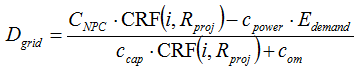# HOMER Pro 3.11

 Navigation: Glossary Break-even Grid Extension DistanceType: Output Variable Units: km Symbol: Dgrid

The break-even grid extension distance is the distance from the grid that makes the net present cost of extending the grid equal to the net present cost of the stand-alone system. Farther away from the grid, the stand-alone system is optimal. Nearer to the grid, grid extension is optimal.

HOMER calculates the break-even grid extension distance using the following equation:where: CNPC = total net present cost of the stand-alone power system [\$] CRF() i Rproj = project lifetime [yr] Edemand = total annual electrical demand (primary plus deferrable) [kWh/yr] cpower = cost of power from the grid [\$/kWh] ccap = capital cost of grid extension [\$/km] com = O&M cost of grid extension [\$/yr/km]